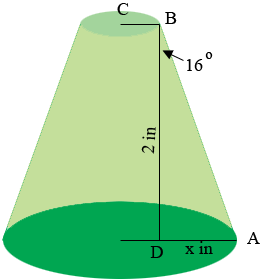SEARCH HOMEMath Central Quandaries & QueriesQuestion from Brandon: Need a formula to find missing large Dia of a truncated cone where the small dia =.19" Height= 2" and taper =16degHi Brandon,

I drew a diagram of what I see from your description.Since the angle $BDA$ is a right angle

$\tan(16^o) = \frac{x}{2} \mbox{ and hence } x = 2 \times \tan(16^o) = 2 \times 0.2867 = 0.5735.$

Hence the diameter of the circular base of my truncated cone is $0.19 + 2 \times 0.5735 = 1.34$ inches.

I hope this helps,
HarleyMath Central is supported by the University of Regina and The Pacific Institute for the Mathematical Sciences.